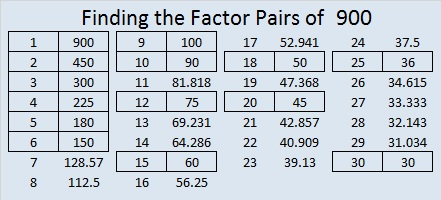# 900 Pick Your Pony. Who’ll Win This Amount of Factors Horse Race?

I really like this rhyme that I saw for the first time this week (even though it’s all over the net):

Hey diddle diddle, the median’s the middle,
You add then divide for the mean.
The mode is the one that appears the most,
And the range is the difference between.

All of the numbers from 801 to 900 have at least 2 factors, but no more than 32 factors. 32 – 2 = 30, so 30 is the range of the amount of factors.

There are 100 numbers from 801 to 900. If you list the amount of factors for each number, then arrange those amounts from smallest to largest, the amounts that will appear in the 50th and 51st spots will both be 6. That means that 6 is the median amount of factors. If we had different amounts in the 50th and 51st spots, we would average the two amounts together to get the median.

If you add up the amounts of factors that the numbers from 801 to 900 have, you will get 794. If you divide 794 by 100, the number of entries, then you will know that 7.94 is the mean amount of factors.

What about the mode? Which amount of factors appears the most? That’s why we are having a Horse Race, to see if more numbers have 2 factors, 3 factors, 4 factors, or a different amount of factors. So pick your pony. We’ll see which amount wins, and we’ll find out what the mode is at the same time.

The contenders are these amounts: 2, 3, 4, 6, 8, 10, 12, 14, 16, 18, 20, 24, 27, 32.

I should tell you that only perfect squares can have an odd amount of factors, so you probably don’t want to pick an odd amount.Here are some interesting facts about the numbers from 801 to 900 that might help you decide which pony to pick.

• We had the smallest two consecutive numbers with exactly 12 factors: (819, 820)
• We had the fourth prime decade: (821, 823, 827, 829). All four of those numbers are prime numbers and have exactly two factors.
• We had five consecutive numbers whose square roots can be reduced: (844, 845, 846, 847, 848). Three of those numbers had 6 factors, one had 10, and one had 12.
• We also had 840, the smallest number with exactly 32 factors
• 900 is the smallest number with exactly 27 factors. Coincidentally, the amount that is the mode will appear 27 times.

As the following table shows, there are 42 integers from 801 to 900 that have square roots that can be simplified. 42 is more than any previous set of 100 numbers has given us. Even still we are still holding close to just under 40% of integers having square roots that can be simplified.Okay. If you’ve picked your pony, NOW you can watch the Horse Race:

make science GIFs like this at MakeaGif
Hmm…

The race was exciting for a second or two.

As you can see from the Horse Race the mode is 4. How did your pony do?

Here’s a little more about the number 900:

900 is the sum of the fourteen prime numbers from 37 to 97.

24² + 18² = 900

900 is the hypotenuse of two Pythagorean triples:

• 252-864-900, which is 24² – 18², 2(24)(18), 24² + 18². It is also (7-24-25) times 36.
• 540-720-900, which is (3-4-5) times 180.

900 is the sum of the interior angles of a heptagon (seven-sided polygon).

• 900 is a composite number and a perfect square.
• Prime factorization: 900 = 2 × 2 × 3 × 3 × 5 × 5, which can be written 900 = 2² × 3² × 5²
• The exponents in the prime factorization are 2, 2 and 2. Adding one to each and multiplying we get (2 + 1)(2 + 1)(1 + 1) = 3 × 3 × 3 = 27. Therefore 900 has exactly 27 factors.
• Factors of 900: 1, 2, 3, 4, 5, 6, 9, 10, 12, 15, 18, 20, 25, 30, 36, 45, 50, 60, 75, 90, 100, 150, 180, 225, 300, 450, 900
• Factor pairs: 900 = 1 × 900, 2 × 450, 3 × 300, 4 × 225, 5 × 180, 6 × 150, 9 × 100, 10 × 90, 12 × 75, 15 × 60, 18 × 50, 20 × 45, 25 × 36, or 30 × 30
• Taking the factor pair with the largest square number factor, we get √900 = (√30)(√30) = 30.This site uses Akismet to reduce spam. Learn how your comment data is processed.